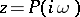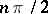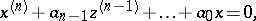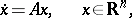# Mikhailov criterion

(diff) ← Older revision | Latest revision (diff) | Newer revision → (diff)

All roots of a polynomialwith real coefficients have strictly negative real part if and only if the complex-valued functionof a real variabledescribes a curve (the Mikhailov hodograph) in the complex-plane which starts on the positive real semi-axis, does not hit the origin and successively generates an anti-clockwise motion throughquadrants. (An equivalent condition is: The radius vector, asincreases fromto, never vanishes and monotonically rotates in a positive direction through an angle.)

This criterion was first suggested by A.V. Mikhailov . It is equivalent to the Routh–Hurwitz criterion; however, it is geometric in character and does not require the verification of determinant inequalities (see , ). The Mikhailov criterion gives a necessary and sufficient condition for the asymptotic stability of a linear differential equation of order,with constant coefficients, or of a linear systemwith a constant matrix, the characteristic polynomial of which is(see ).

Mikhailov's criterion is one of the frequency criteria for the stability of linear systems of automatic control (closely related to, for example, the Nyquist criterion). A generalization of Mikhailov's criterion is known for systems of automatic control with delay, for impulse systems (see ), and there is also an analogue of Mikhailov's criterion for non-linear control systems (see ).

How to Cite This Entry:
Mikhailov criterion. Encyclopedia of Mathematics. URL: http://encyclopediaofmath.org/index.php?title=Mikhailov_criterion&oldid=12540
This article was adapted from an original article by r equation','../w/w097310.htm','Whittaker equation','../w/w097840.htm','Wronskian','../w/w098180.htm')" style="background-color:yellow;">N.Kh. Rozov (originator), which appeared in Encyclopedia of Mathematics - ISBN 1402006098. See original article